# Package update – Plotting multi-objective linear/integer programming models in R

R package gMOIP has been updated to version 1.3.0 and now can plot 3D models too.

The package can make 2D and 3D plots of the polytope of a linear programming (LP), integer linear programming (ILP) model, or mixed integer linear programming (MILP) model with 2 or 3 variables, including integer points, ranges and iso profit curve. Moreover you can also make a plot of the bi-objective criterion space and the non-dominated (Pareto) set for bi-objective LP/ILP/MILP programming models. Figures can be prepared for LaTeX and can automatically be transformed to TikZ using package tikzDevice.

You can install the latest stable release from CRAN:

install.packages("gMOIP")

Alternatively (recommended), install the latest development version from GitHub:

install.packages("devtools")
devtools::install_github("relund/gMOIP",build_vignettes = TRUE)

#' Function for loading missing packages. Install a package from CRAN if not already installed.
#'
#' @param packages String vector with package names
#'
#' @return NULL (invisible)
#' @export
#'
newP <- packages[!(packages %in% installed.packages()[,"Package"])]
if(length(newP)) install.packages(newP, repos = "http://cran.rstudio.com/")
lapply(packages, library, character.only = TRUE)
invisible(NULL)
}
loadPackages("gMOIP")

Next, let us have a look at some examples.

## A bi-objective model with two variables

We define a function for grouping plots of the solution and criterion space (you may just use functions plotPolytope and plotCriterion2D for single plots):

loadPackages("gridExtra")  # to combine two plots into one
plotBiObj2D <- function(A, b, obj,
type = rep("c", ncol(A)),
crit = "max",
faces = rep("c", ncol(A)),
plotFaces = TRUE,
plotFeasible = TRUE,
plotOptimum = FALSE,
labels = "numb",
{
p1 <- plotPolytope(A, b, type = type, crit = crit, faces = faces, plotFaces = plotFaces,
plotFeasible = plotFeasible, plotOptimum = plotOptimum, labels = labels) +
ggplot2::ggtitle("Solution space")
p2 <- plotCriterion2D(A, b, obj, type = type, crit = crit, addTriangles = addTriangles,
ggplot2::ggtitle("Criterion space")
gridExtra::grid.arrange(p1, p2, nrow = 1)
}

Let us define the constraints:

A <- matrix(c(-3,2,2,4,9,10), ncol = 2, byrow = TRUE)
b <- c(3,27,90)

First let us have a look at a LP model (maximize):

obj <- matrix(
c(7, -10, # first criterion
-10, -10), # second criterion
nrow = 2)
plotBiObj2D(A, b, obj, addTriangles = FALSE)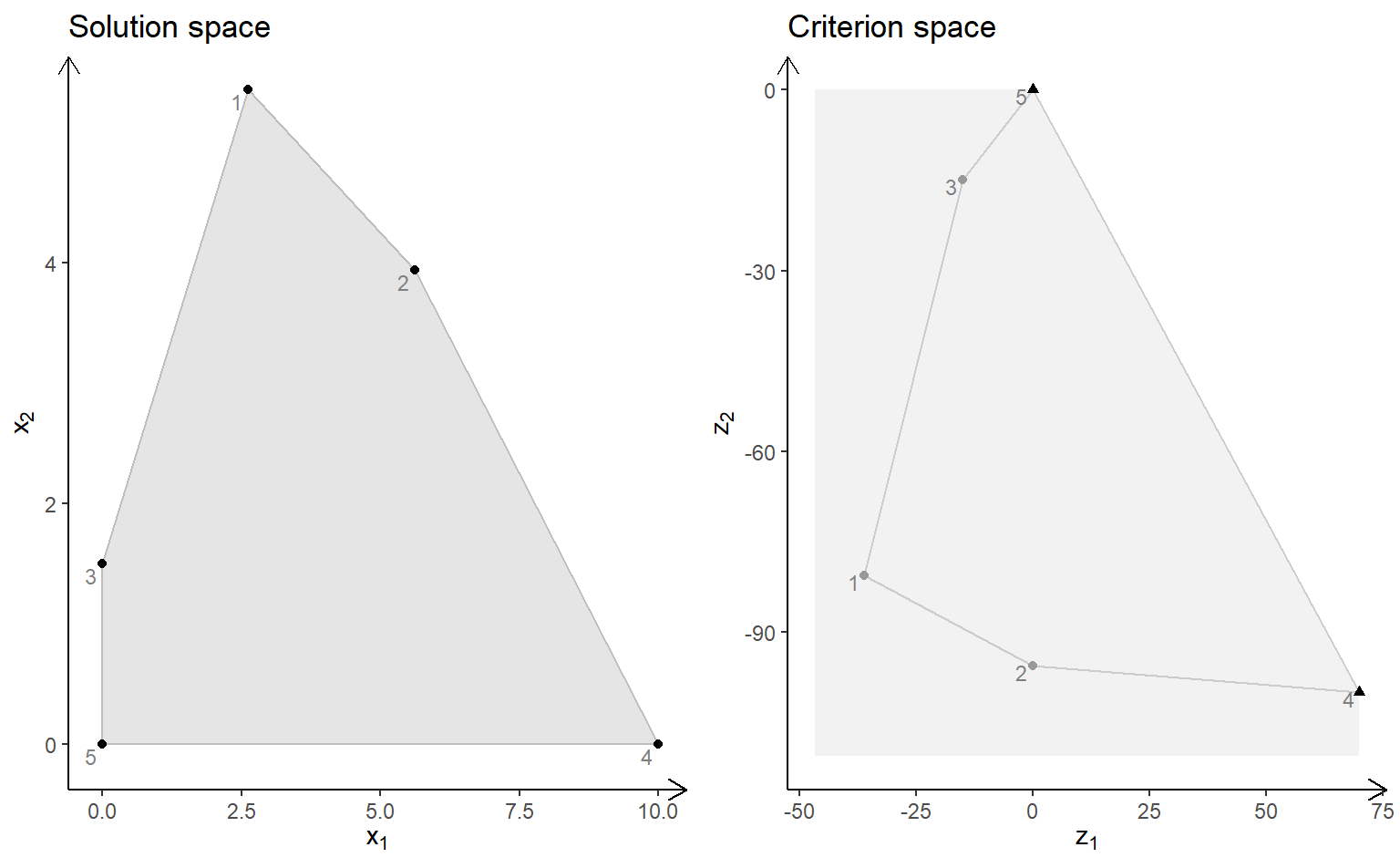Note the non-dominated (Pareto) set consists of all supported extreme non-dominated points (illustrated with triangles) and the line segments between them (supported non-dominated points).

ILP models with different criteria (maximize):

obj <- matrix(c(7, -10, -10, -10), nrow = 2)
plotBiObj2D(A, b, obj, type = rep("i", ncol(A)))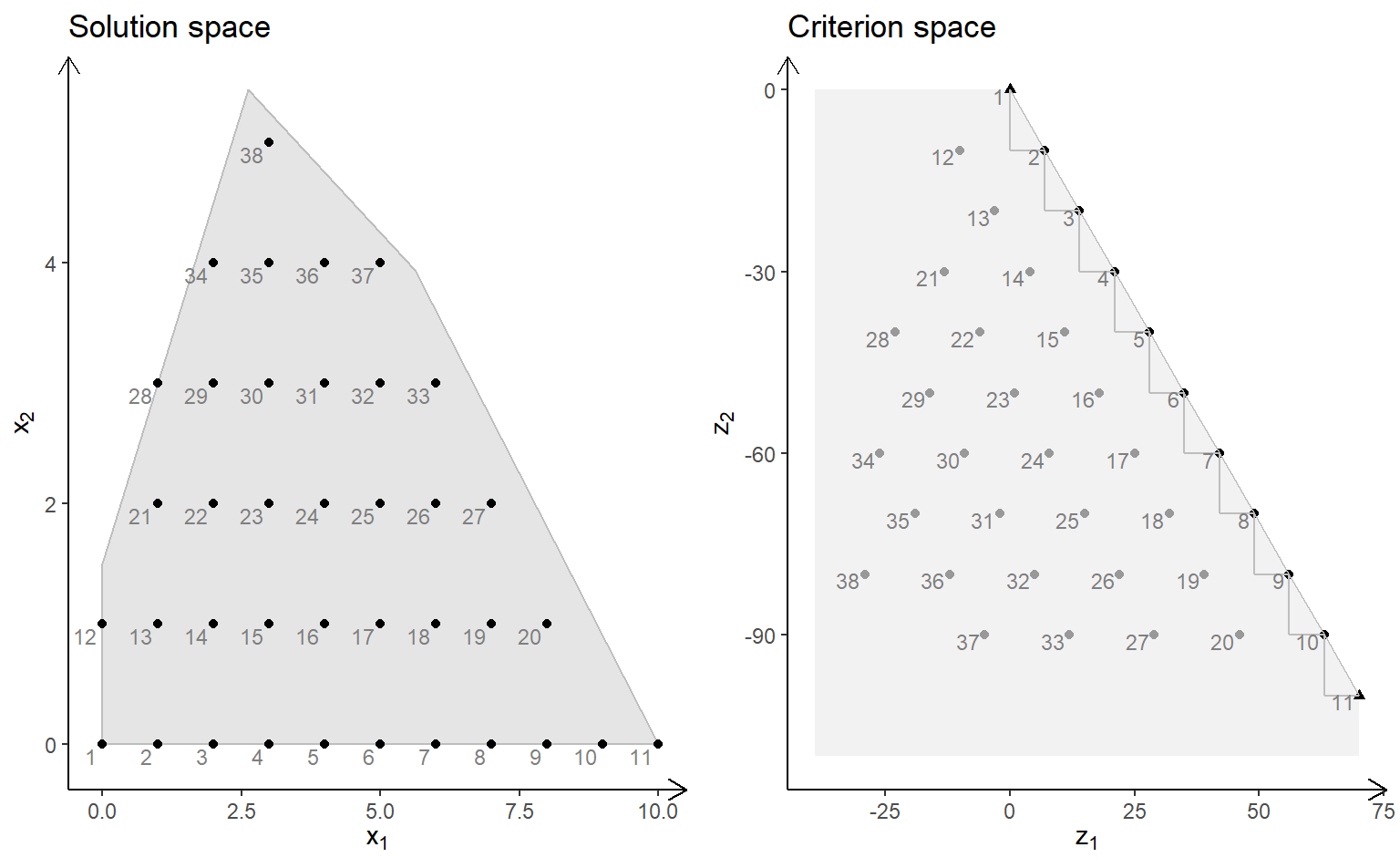obj <- matrix(c(3, -1, -2, 2), nrow = 2)
plotBiObj2D(A, b, obj, type = rep("i", ncol(A)))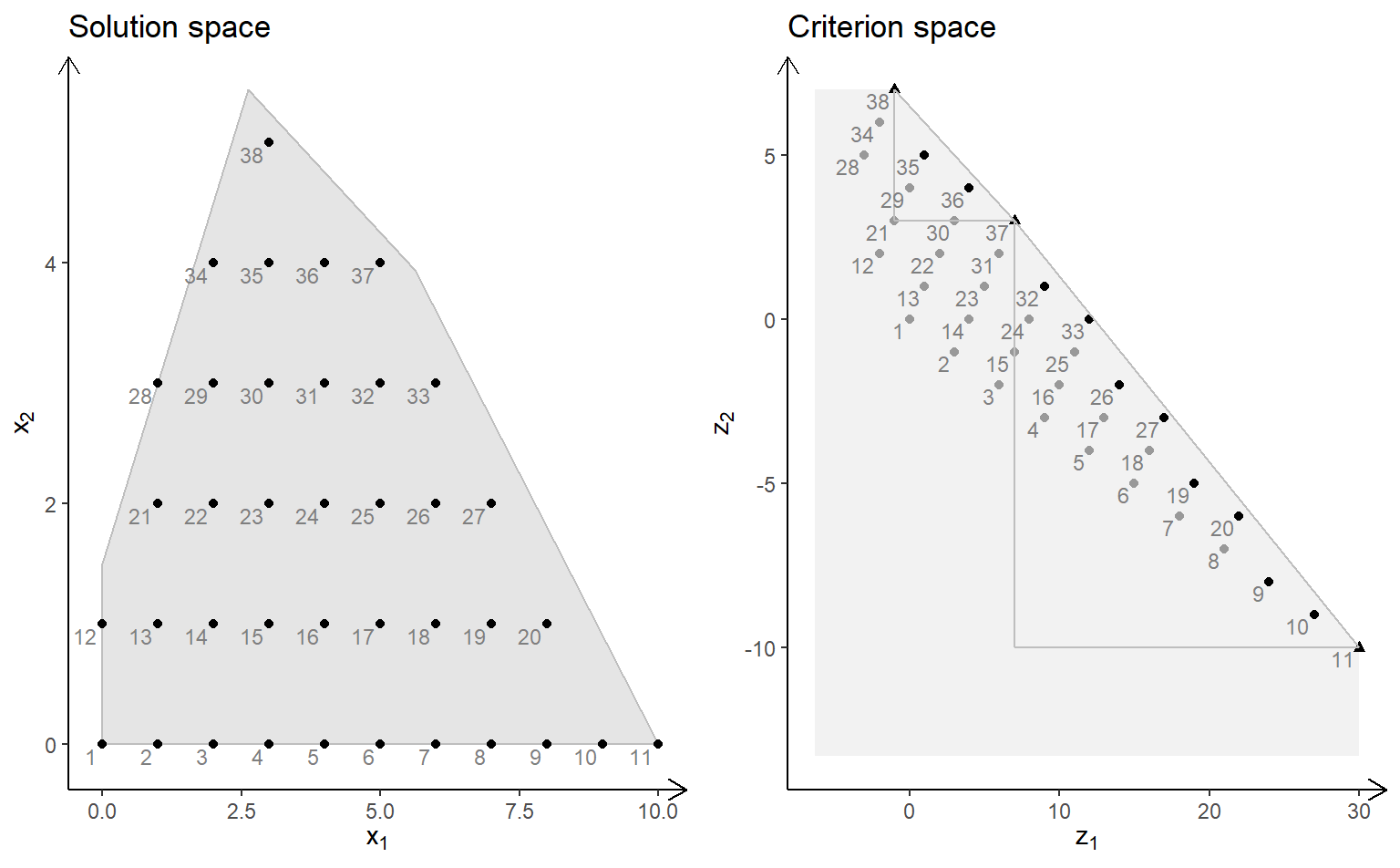obj <- matrix(c(-7, -1, -5, 5), nrow = 2)
plotBiObj2D(A, b, obj, type = rep("i", ncol(A)))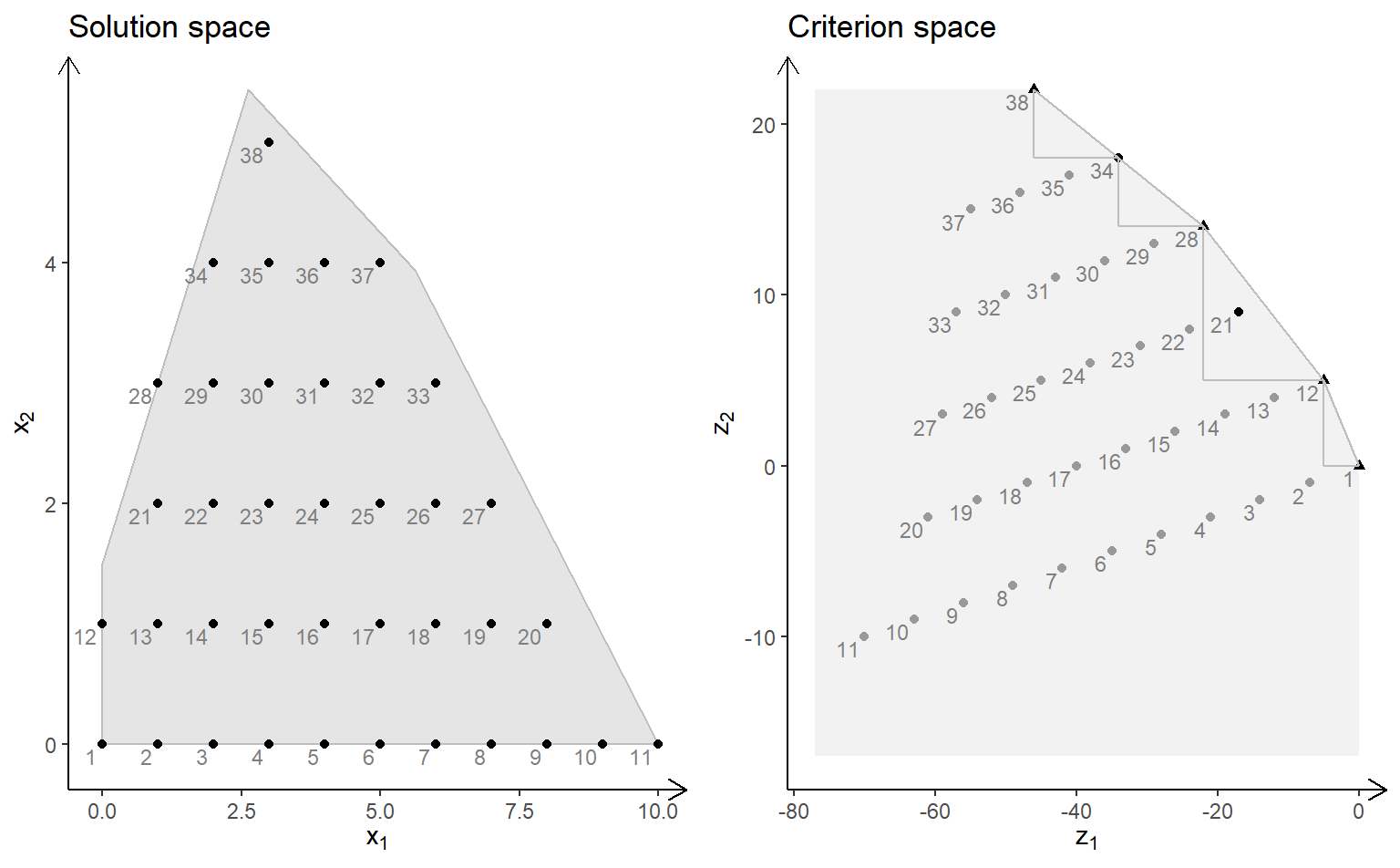obj <- matrix(c(-1, -1, 2, 2), nrow = 2)
plotBiObj2D(A, b, obj, type = rep("i", ncol(A)))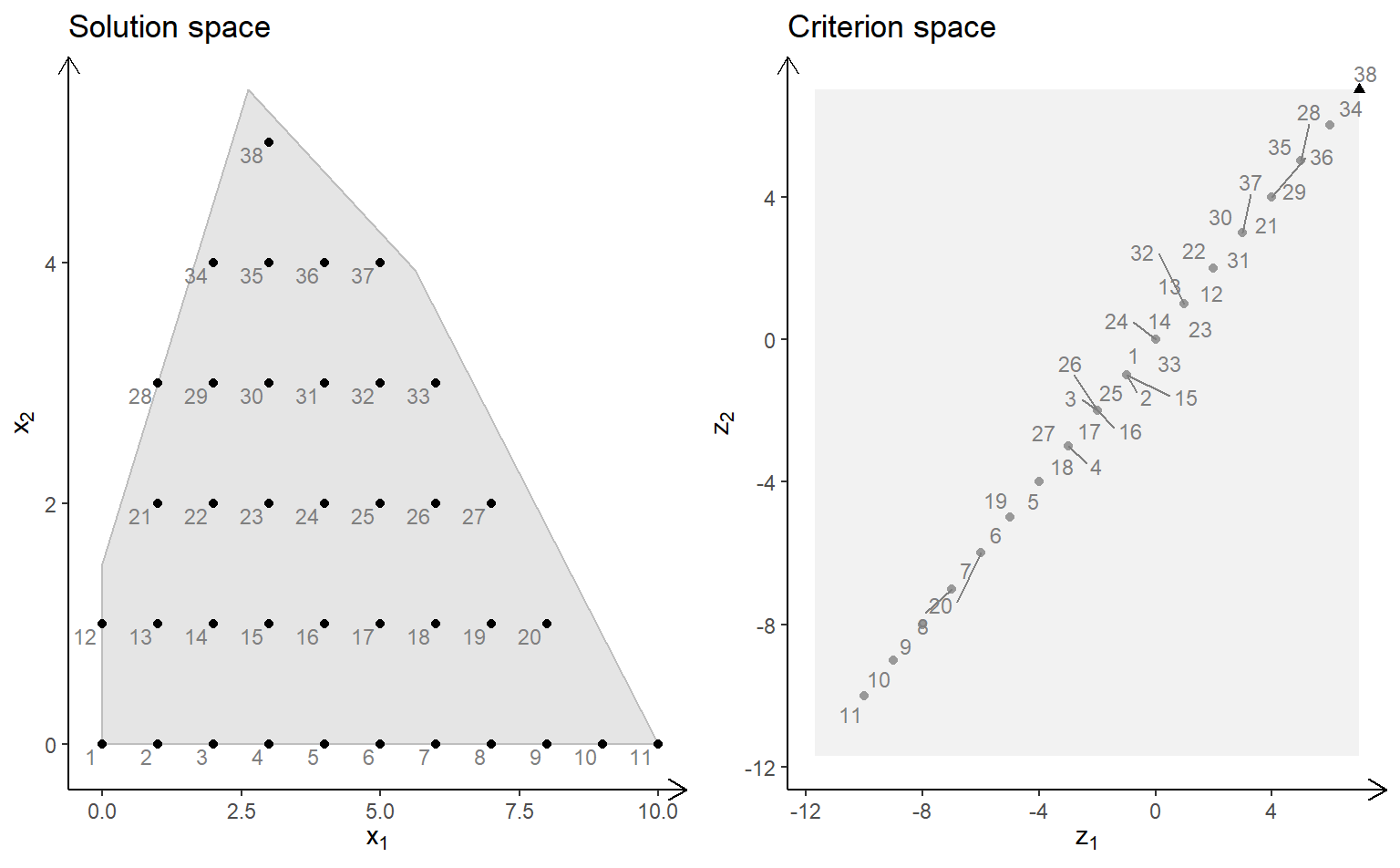Note the non-dominated set consists of all points in black (with shape supported extreme = triangle, supported non-extreme = round, unsupported = round (not on the border)). The triangles drawn using the extreme non-dominated points illustrate areas where unsupported non-dominated points may be found. A point in the solution space is identified in the criterion space using the same number.

ILP models with different criteria (minimize):

obj <- matrix(c(7, -10, -10, -10), nrow = 2)
plotBiObj2D(A, b, obj, type = rep("i", ncol(A)), crit = "min")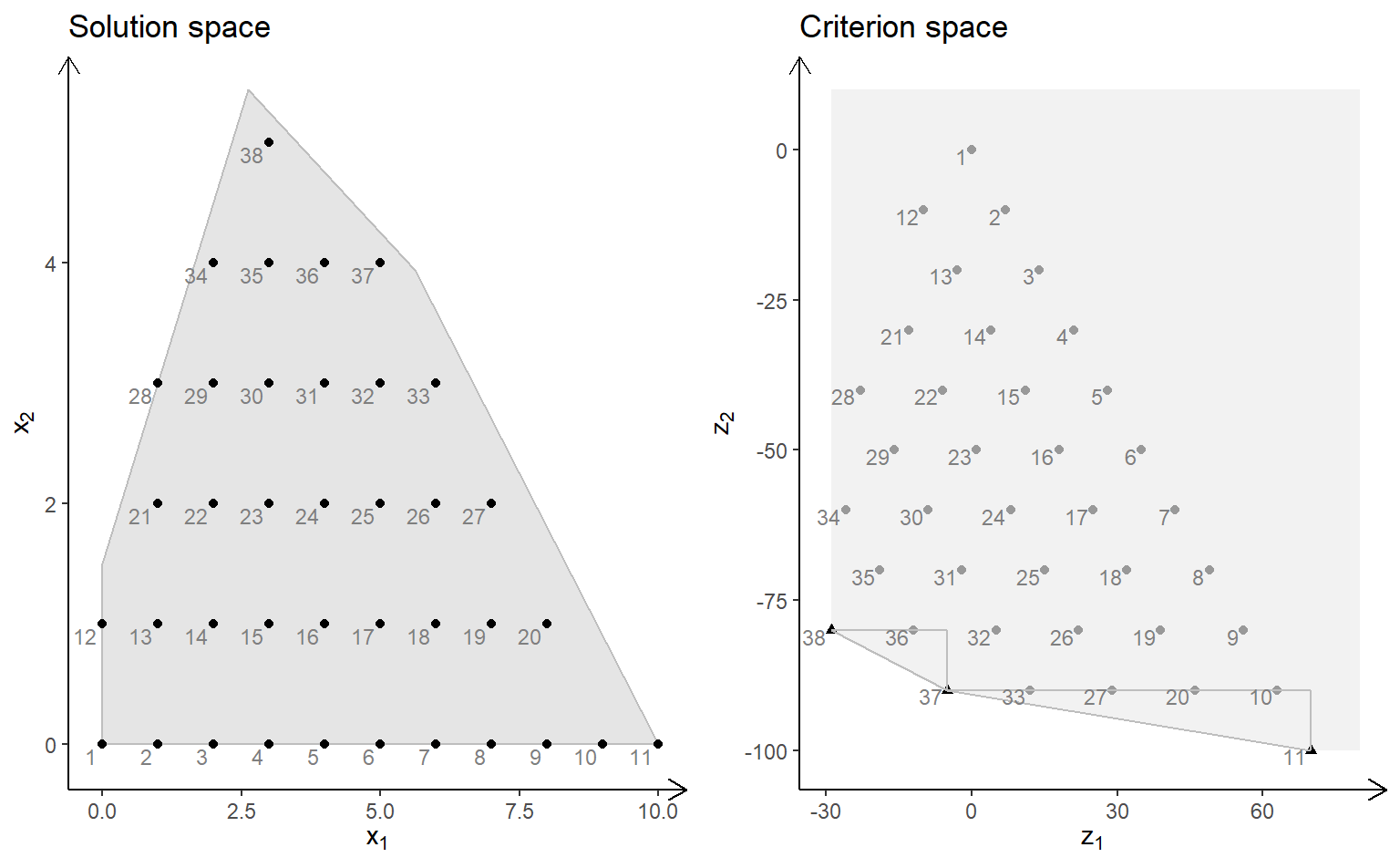obj <- matrix(c(3, -1, -2, 2), nrow = 2)
plotBiObj2D(A, b, obj, type = rep("i", ncol(A)), crit = "min")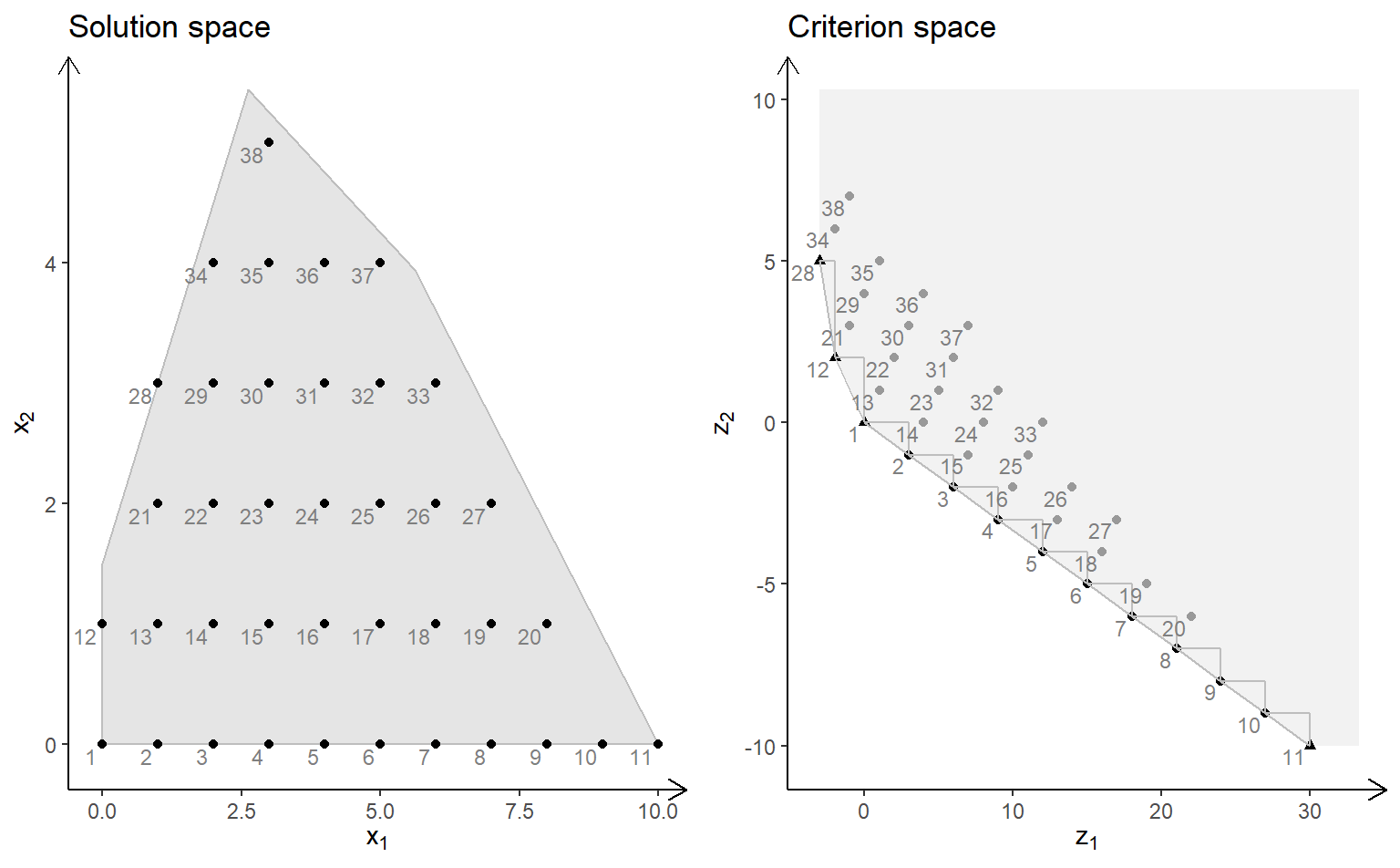obj <- matrix(c(-7, -1, -5, 5), nrow = 2)
plotBiObj2D(A, b, obj, type = rep("i", ncol(A)), crit = "min")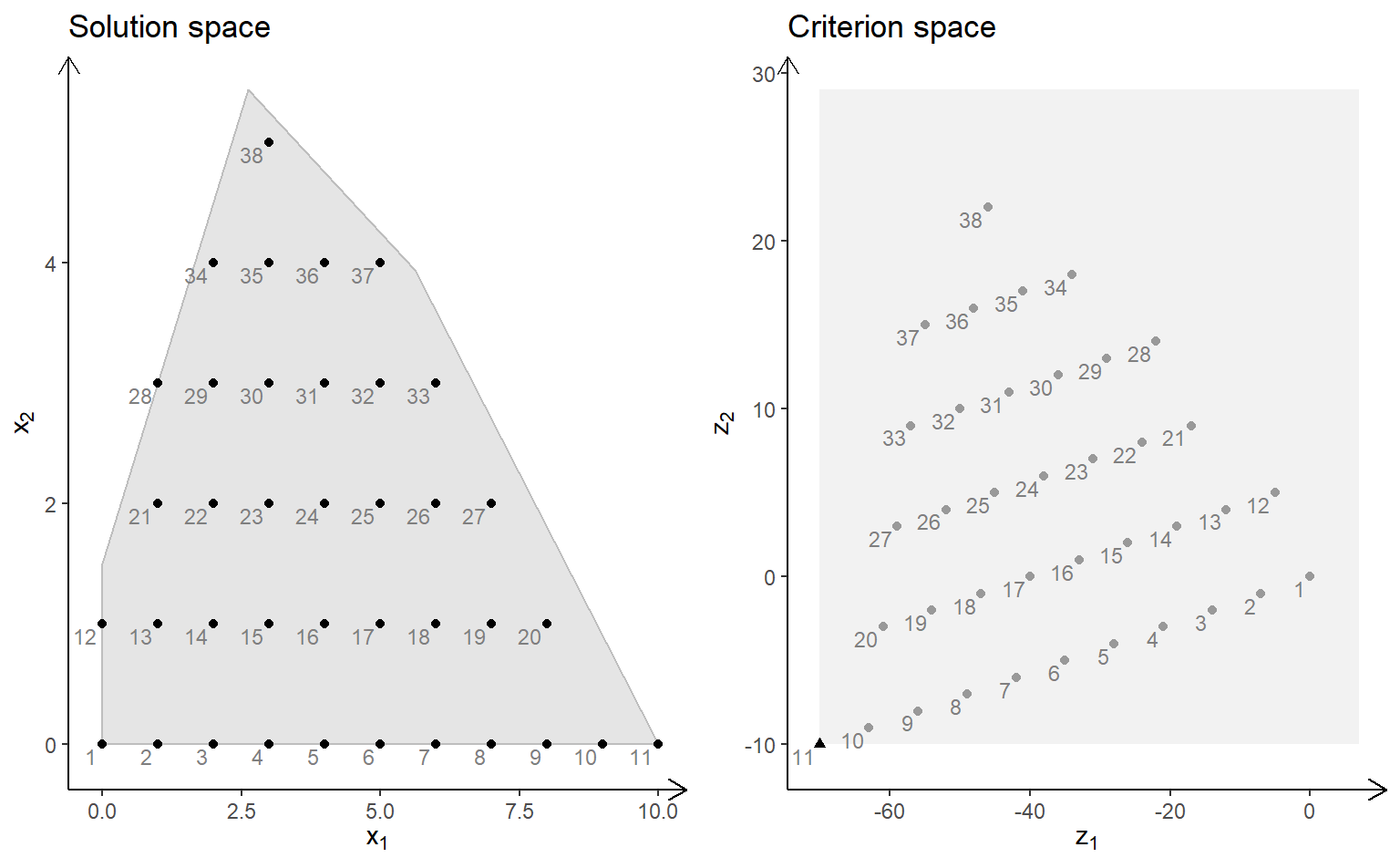obj <- matrix(c(-1, -1, 2, 2), nrow = 2)
plotBiObj2D(A, b, obj, type = rep("i", ncol(A)), crit = "min")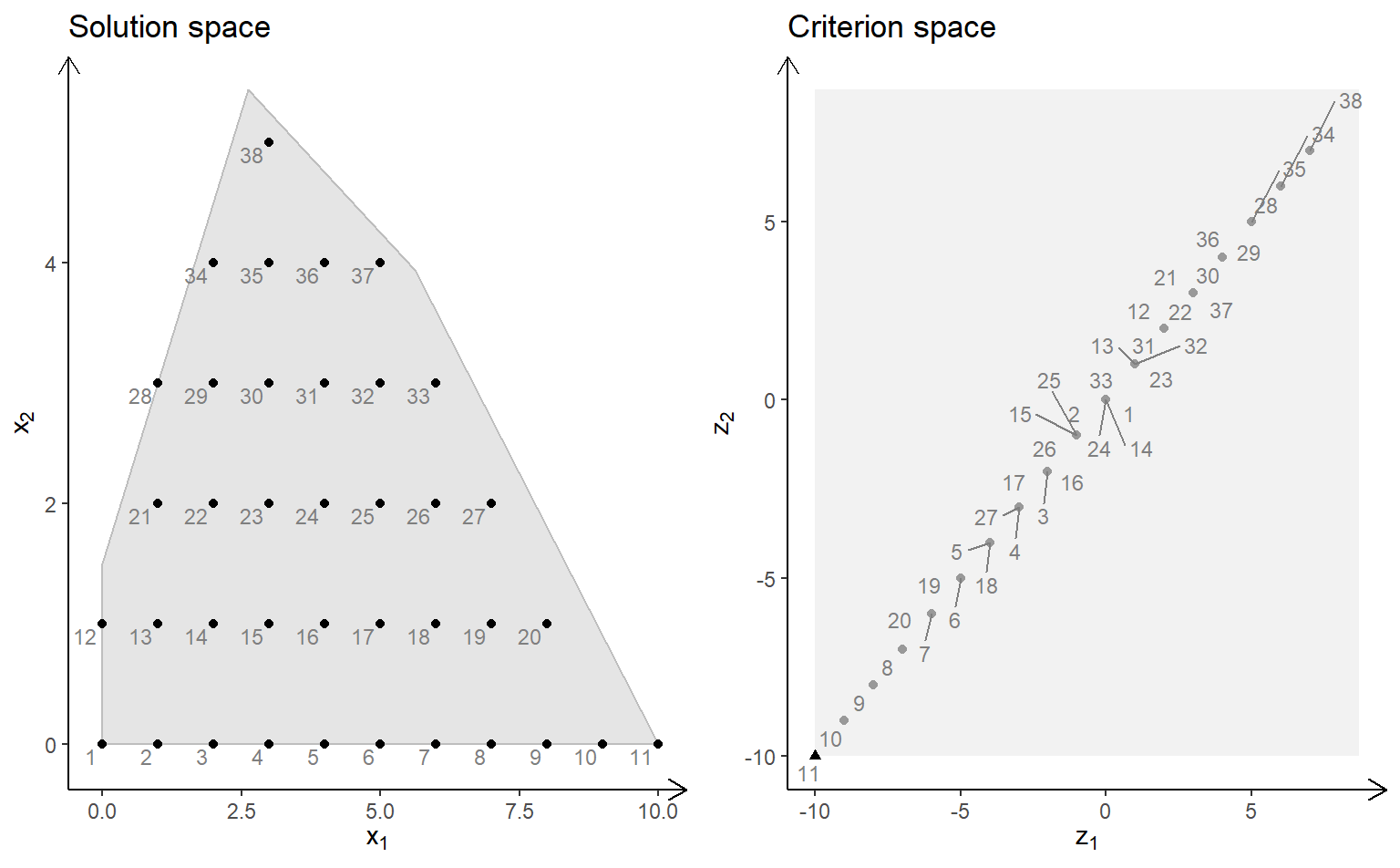MILP model ($x_1$ integer) with different criteria (maximize):

obj <- matrix(c(7, -10, -10, -10), nrow = 2)
plotBiObj2D(A, b, obj, type = c("i", "c"))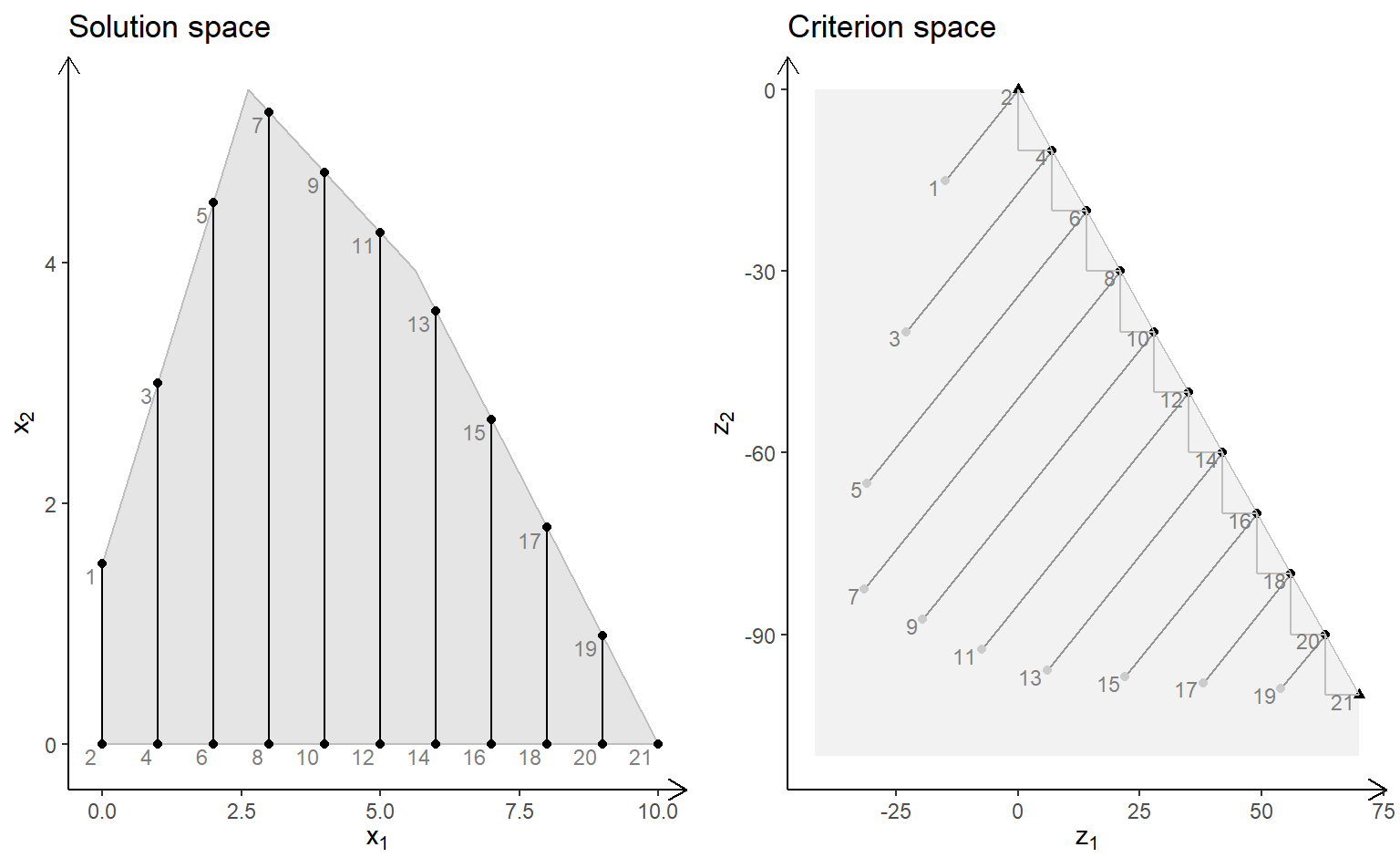obj <- matrix(c(3, -1, -2, 2), nrow = 2)
plotBiObj2D(A, b, obj, type = c("i", "c"))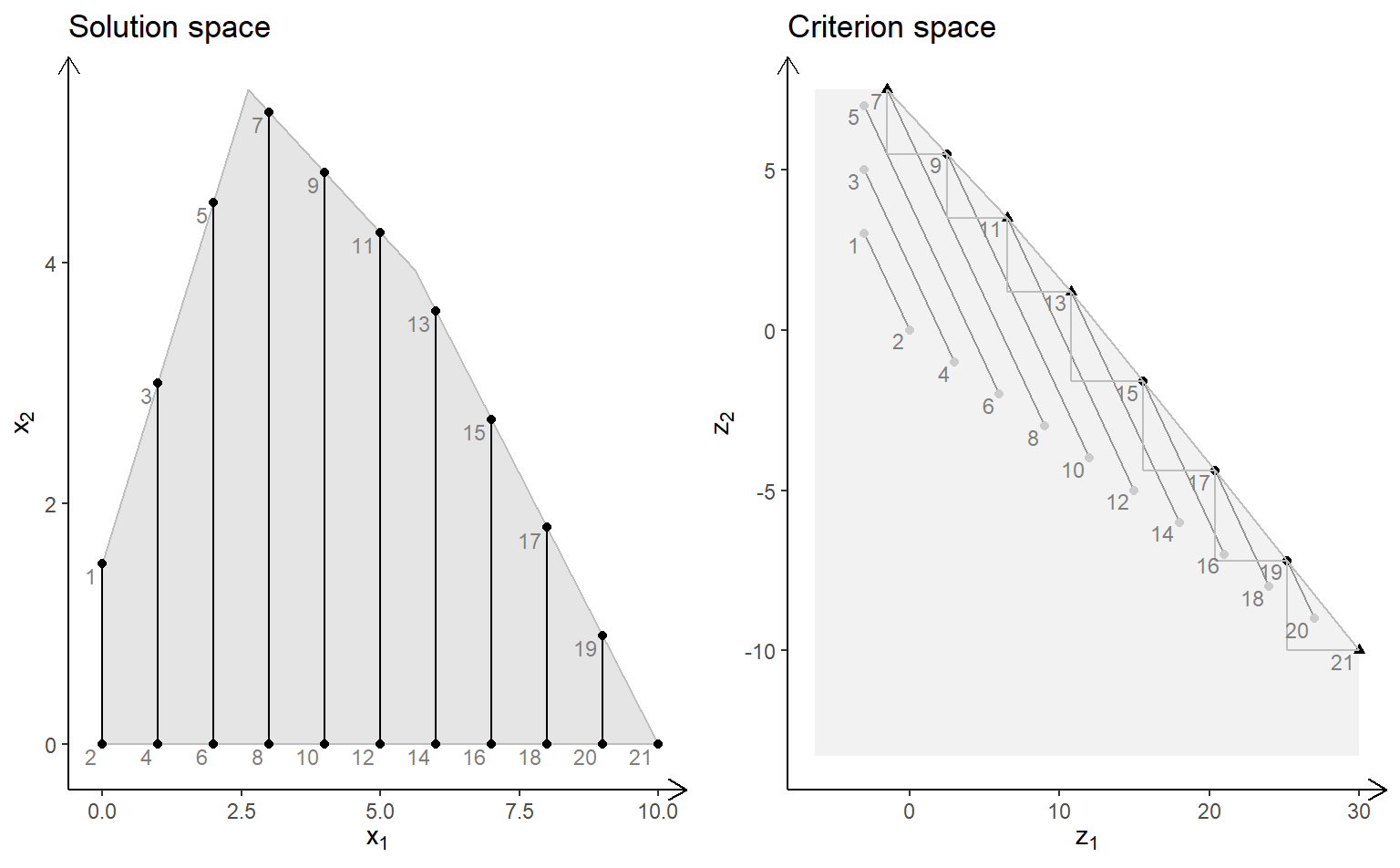obj <- matrix(c(-7, -1, -5, 5), nrow = 2)
plotBiObj2D(A, b, obj, type = c("i", "c"))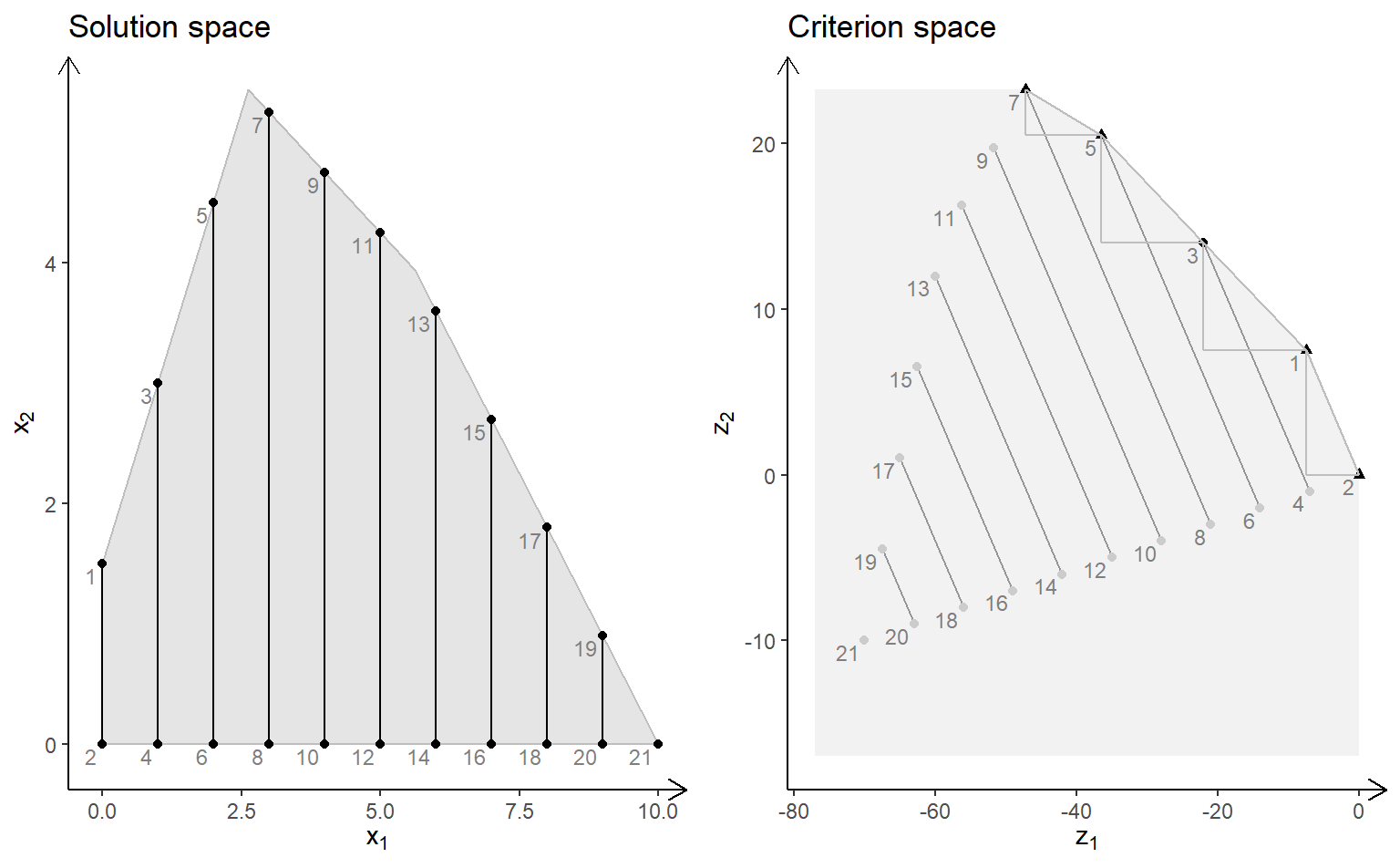obj <- matrix(c(-1, -1, 2, 2), nrow = 2)
plotBiObj2D(A, b, obj, type = c("i", "c"))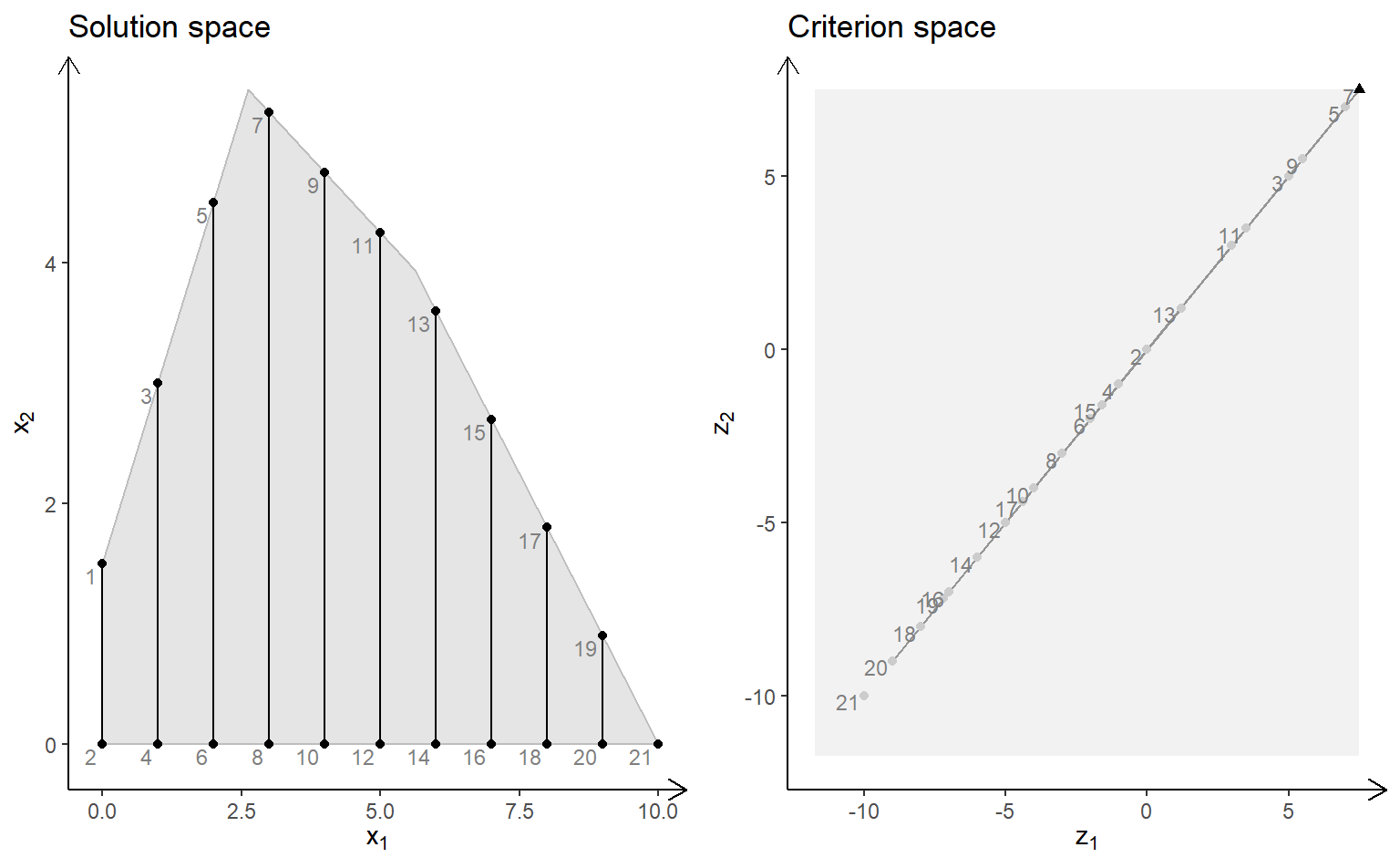Note the solution space now consists to segments and hence the non-dominated set may consist of points and segments (open and closed). Note these segments are not highlighted in the current version of gMOIP.

MILP model ($x_2$ integer) with different criteria (minimize):

##
obj <- matrix(c(7, -10, -10, -10), nrow = 2)
plotBiObj2D(A, b, obj, type = c("c", "i"), crit = "min")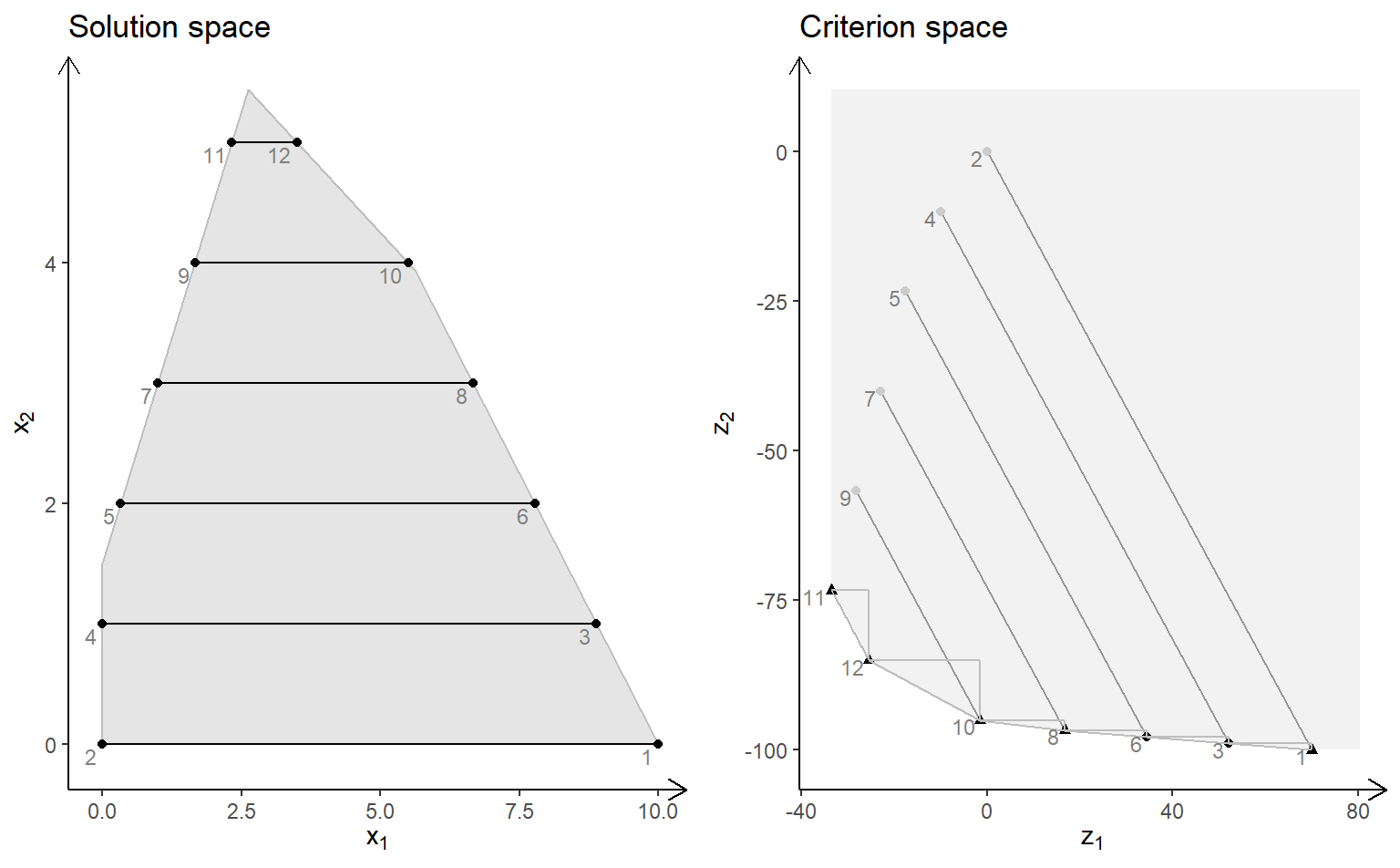obj <- matrix(c(3, -1, -2, 2), nrow = 2)
plotBiObj2D(A, b, obj, type = c("c", "i"), crit = "min")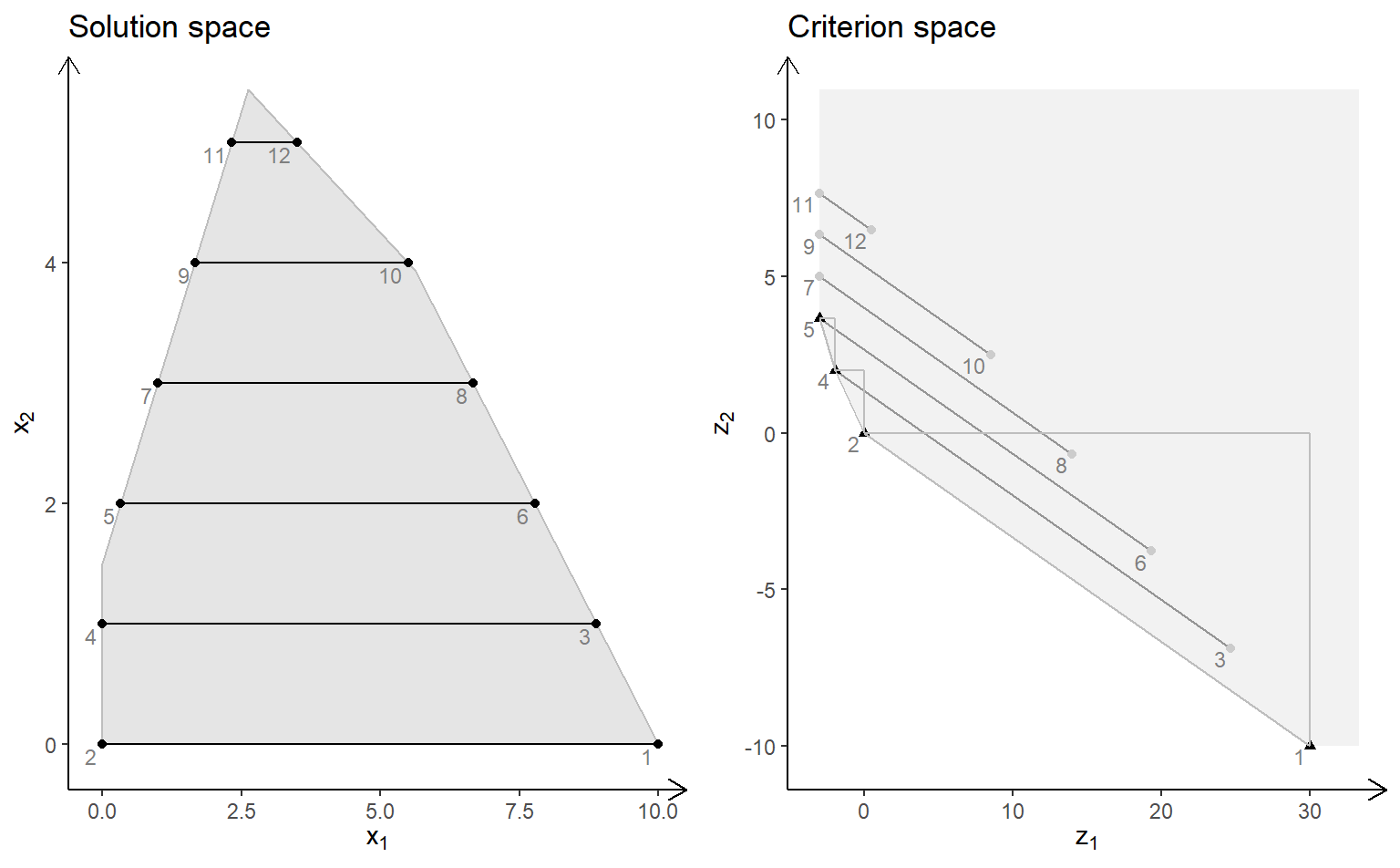obj <- matrix(c(-7, -1, -5, 5), nrow = 2)
plotBiObj2D(A, b, obj, type = c("c", "i"), crit = "min")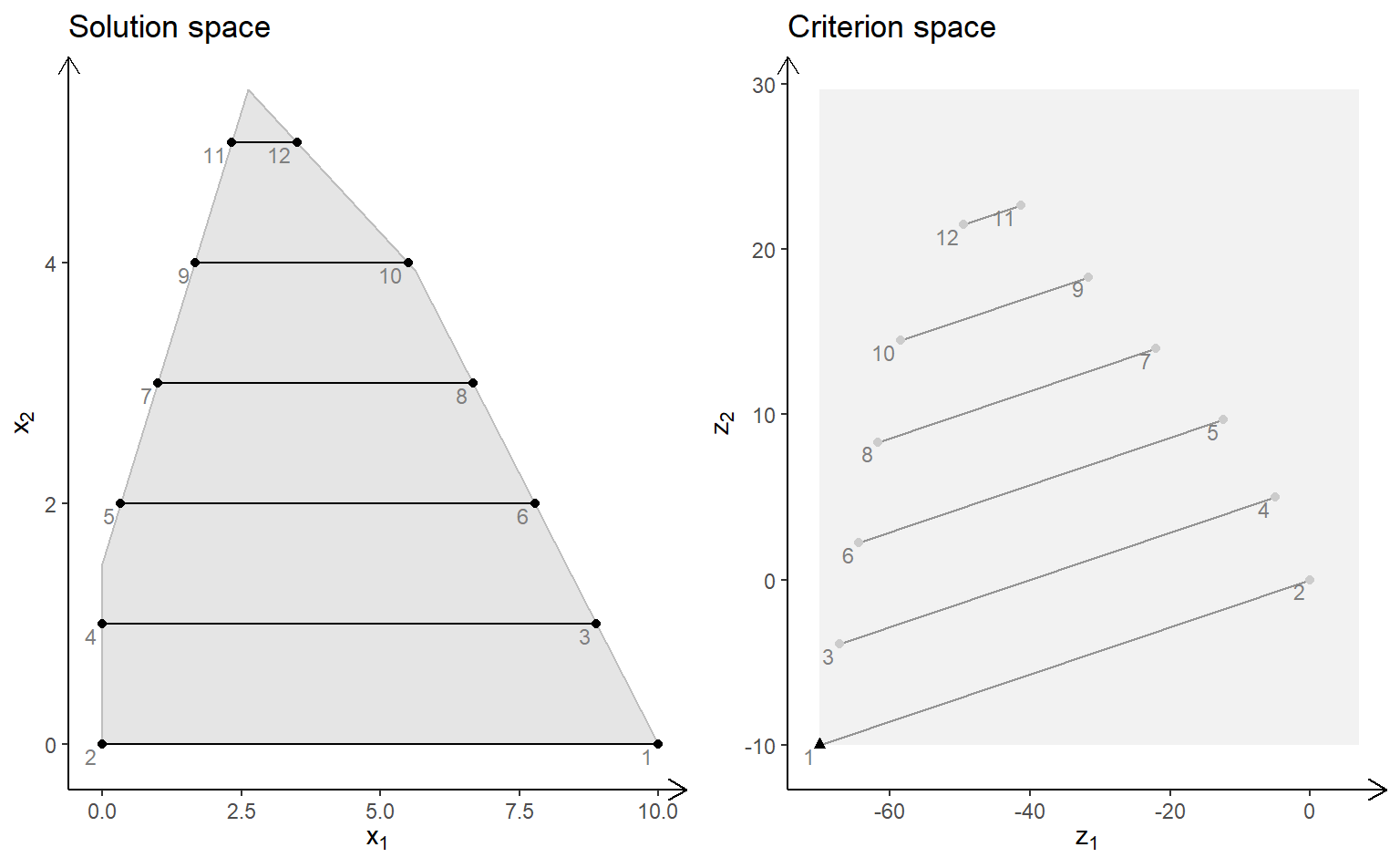obj <- matrix(c(-1, -1, 2, 2), nrow = 2)
plotBiObj2D(A, b, obj, type = c("c", "i"), crit = "min")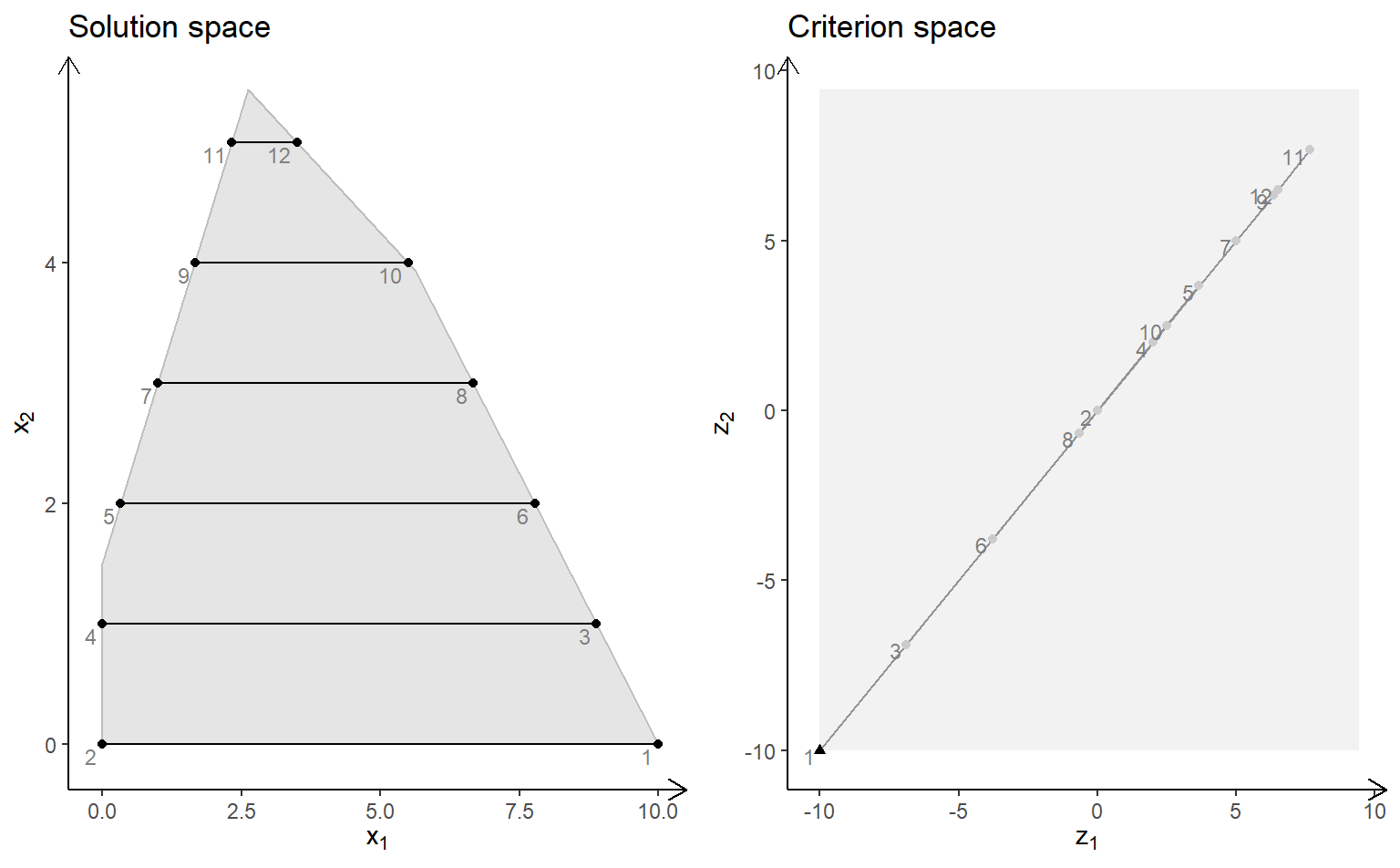## A bi-objective model with three variables

We define functions for plotting the solution and criterion space:

plotSol <- function(A, b, type = rep("c", ncol(A)),
faces = rep("c", ncol(A)),
plotFaces = TRUE, labels = "numb")
{
loadView(v = view, close = F, zoom = 0.75)
plotPolytope(A, b, type = type, faces = faces, labels = labels, plotFaces = plotFaces,
argsTitle3d = list(main = "Solution space"))
}

plotCrit <- function(A, b, obj, crit = "min", type = rep("c", ncol(A)), addTriangles = TRUE,
labels = "numb")
{
plotCriterion2D(A, b, obj, type = type, crit = crit, addTriangles = addTriangles,
labels = labels) +
ggplot2::ggtitle("Criterion space")
}

We define the model $\max \{cx | Ax \leq b\}$ (could also be minimized) with three variables:

Ab <- matrix( c(
1, 1, 2, 5,
2, -1, 0, 3,
-1, 2, 1, 3,
0, -3, 5, 2
), nc = 4, byrow = TRUE)
A <- Ab[,1:3]
b <- Ab[,4]
obj <- matrix(c(1, -6, 3, -4, 1, 6), nrow = 2)

We load the preferred view angle for the 3D window:

view <- matrix( c(-0.452365815639496, -0.446501553058624, 0.77201122045517, 0, 0.886364221572876,
-0.320795893669128, 0.333835482597351, 0, 0.0986008867621422, 0.835299551486969,
0.540881276130676, 0, 0, 0, 0, 1), nc = 4)
loadView(v = view)

LP model (solution space – interactive plot):

plotSol(A, b)

plotCrit(A, b, obj, addTriangles = FALSE)